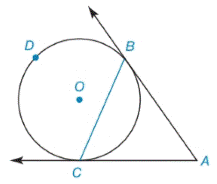Chapter 6.2, Problem 8E### Elementary Geometry for College St...

6th Edition
Daniel C. Alexander + 1 other
ISBN: 9781285195698

#### Solutions

Chapter
Section### Elementary Geometry for College St...

6th Edition
Daniel C. Alexander + 1 other
ISBN: 9781285195698
Textbook Problem
1 views

# Given: A B → and A C → are tangent to ⊙ O , m B C ⌢ = 126 ° Find: a) m ∠ A b) m ∠ A B C c) m ∠ A C BTo determine

(a)

To find:

mA when AB and AC are tangent to O, mBC=126°.

Explanation

Theorem:

The measure of an angle formed when two intersecting tangents, then the measure of angle is one-half the difference of the measures of the two intercepted arc.

Calculation:

mA when AB and AC are tangent to O, mBC=126°

mBC+mBDC=360°126°+mBDC=360°

To determine

(b)

To find:

mABC when AB and AC are tangent to O, mBC=126°.

To determine

(c)

To find:

mACB when AB and AC are tangent to O, mBC=126°.

### Still sussing out bartleby?

Check out a sample textbook solution.

See a sample solution

#### The Solution to Your Study Problems

Bartleby provides explanations to thousands of textbook problems written by our experts, many with advanced degrees!

Get Started

#### Calculate y'. 41. y=x+1(2x)5(x+3)7

Single Variable Calculus: Early Transcendentals, Volume I

#### 0 1 ∞

Study Guide for Stewart's Multivariable Calculus, 8th

#### A definite integral for the area of the region bounded by y = 2 − x2 and y = x2 is:

Study Guide for Stewart's Single Variable Calculus: Early Transcendentals, 8th